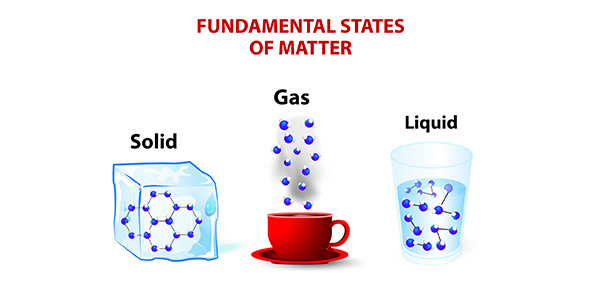# 7 States Of Matter

12 Questions | Total Attempts: 574SettingsYear 7 Science test in the area of states of matter, particle theory and properties of water

• 1.
All matter is made up of tiny particles.
• A.

True

• B.

False

• 2.
Liquids do not move well because their particles are held together strongly.
• A.

True

• B.

False

• 3.
Oxygen is an example of a gas.
• A.

True

• B.

False

• 4.
You need mass and weight to calculate the density of an substance.
• A.

True

• B.

False

• 5.
The skin that is created on the surface of water is called buoyancy.
• A.

True

• B.

False

• 6.
Forces in science can be represented by ____________________.
• 7.
A force can be a
• A.

Push

• B.

Pull

• C.

Twist

• D.

All of the above

• 8.
When water changes from a solid to a liquid the molecules
• A.

Expand

• B.

Contract

• C.

Shrink

• D.

• 9.
When a liquid changes to a gas this process is occuring?
• A.

Condensation

• B.

Evaporation

• C.

Freezing

• D.

Melting

• 10.
If an object is less dense than water, it will sink in water.
• A.

True

• B.

False

• 11.
Choose the correct descriptions for a gas:
• A.

Their particles are not held together at all.

• B.

Their particles can roll over one another.

• C.

Their particles are free to move.

Related TopicsBack to top